# Texas Go Math Grade 3 Lesson 9.4 Answer Key Partial Products

Refer to our Texas Go Math Grade 3 Answer Key Pdf to score good marks in the exams. Test yourself by practicing the problems from Texas Go Math Grade 3 Lesson 9.4 Answer Key Partial Products.

## Texas Go Math Grade 3 Lesson 9.4 Answer Key Partial Products

Essential Question
How can you model and record the products of 2-digit and 1-digit numbers?
We can  model and record the products of 2-digit and 1-digit numbers by different ways like arranging them vertically and doing multiplication or expanded form.

Explanation:
Way:1:
Step I: Arrange the numbers vertically.

Step II: First multiply the digit at the ones place by 2.
2 × 4 = 8 ones.

Step III: Now multiply the digit at the tens place by 2.
2 × 3 = 6 tens.

Way:2. Multiply 20 by 3 by using expanded form

Solution:

20         →                           2 tens + 0 ones

×    3         →                                       ×      3

6 tens + 0 ones

= 60 + 0

= 60

Therefore, 20 × 3 = 60

Unlock the Problem
For a field trip to the science center, there are 4 buses from Maddie’s school with 16 students on each bus. How many students are going on the field trip?
4 × 16 = ___

When you multiply the tens and the ones separately and then add the products, you are finding partial products.

One Way
Make an array with base-ten blocks to model the problem.

Step 1
Use 1 ten 6 ones to show 16. Make 4 rows of l6 to show 4 × 16.Step 2
Combine the tens and the ones to find the product.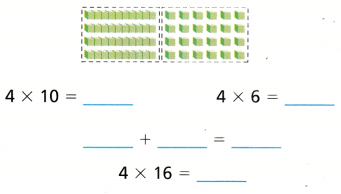Another Way
Draw an array on grid paper.So, ___ students are going on the field trip.
Number of buses from Maddie’s school = 4.
Number of students on each bus = 16.
Number of students are going on the field trip = Number of buses from Maddie’s school  × Number of students on each bus
= 4 × 16
= (4 × 10) + (4 × 6)
= 40 + 24
= 64.
One Way: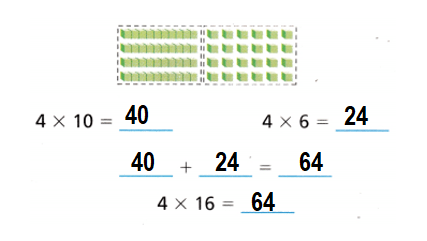Another way: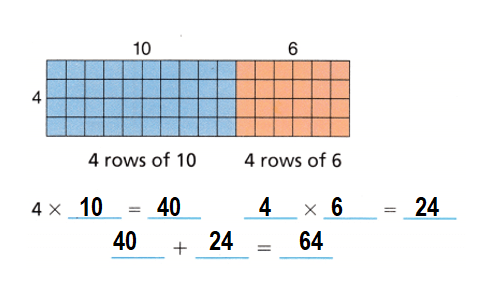Connect Record Multiplication.
You can use what you have learned about the Distributive Property to find products of 2-digit and 1-digit numbers.Shade the model to show each step.Product: 23 × 6 = 138.Explanation:
23 × 6 = ???
Multiply the tens :
23 × 6 = 120.
Multiply the ones :
23 × 6 = 18.
Product:
23 × 6 = 120 + 18 = 138.

Math Talk
Mathematical Processes
Why you record the tens first in step 1.
We record tens place in step 1 because the place value of it highest.

Explanation:
Tens place first we multiply because its the highest place and covers both ones place also.

Share and Show
Question 1.
Model 5 × 18 with base-ten blocks or grid paper. Use paper and pencil to record your multiplication.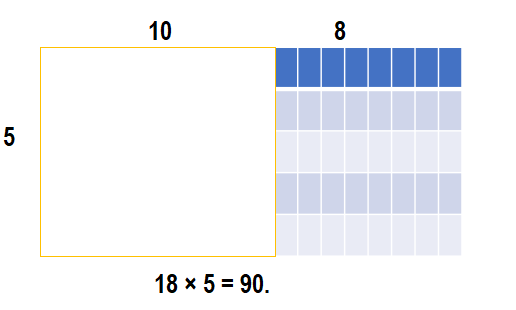Explanation:
Number of rows = 5.
Number of columns = 18.
Product = Number of rows  × Number of columns
= 5 × 18
= (5 × 10) + (5 × 8)
= 50 + 40
= 90.

Find the product.

Question 2.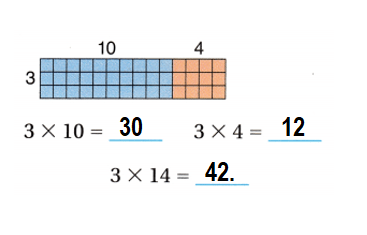Explanation:
Multiply the number of rows:
3 × 10 = 30.
3 × 4 = 12
3 × (10 + 4) = 30 + 12
3 × 14 = 42.

Question 3.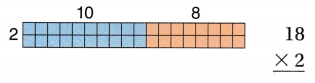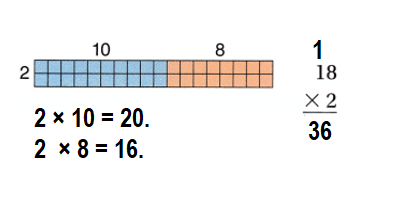Explanation:
Multiply the number of rows:
2 × 10 = 20.
2 × 8 = 16
2 × (20 + 8) = 20 + 16
2 × 18 = 36.

Find the product. Use base-ten blocks or draw an array to help.

Question 4.
14 × 4
Product: 14 × 4 = 56.Explanation:
Number of rows = 4.
Number of columns = 14.
Product = Number of rows  × Number of columns
= 4 × 14
= (4 × 10) + (4 × 4)
= 40  + 16
= 56.

Question 5.
17 × 3
Product: 17 × 3 = 51.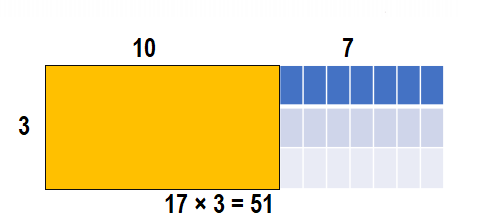Explanation:
Number of rows = 3.
Number of columns = 17.
Product = Number of rows  × Number of columns
= 3 × 17
= (3× 10) + (3 × 7)
= 30 + 21
= 51.

Question 6.
52 × 6
Product: 52 × 6 = 312.Explanation:
Number of rows = 6.
Number of columns = 52.
Product = Number of rows  × Number of columns
= 6 × 52
= (6 × 10) + (6 × 10) + (6 × 10) + (6 × 10) + (6 × 10) + (6 × 2)
= 60 + 60 + 60 + 60 + 60 + 12
= 120 + 60 + 60 + 60 + 12
= 180 + 60 + 60 + 12
= 240 + 60 + 12
= 300 + 12
= 312.
= 90.

Question 7.
41 × 7
Product: 41 × 7 = 287.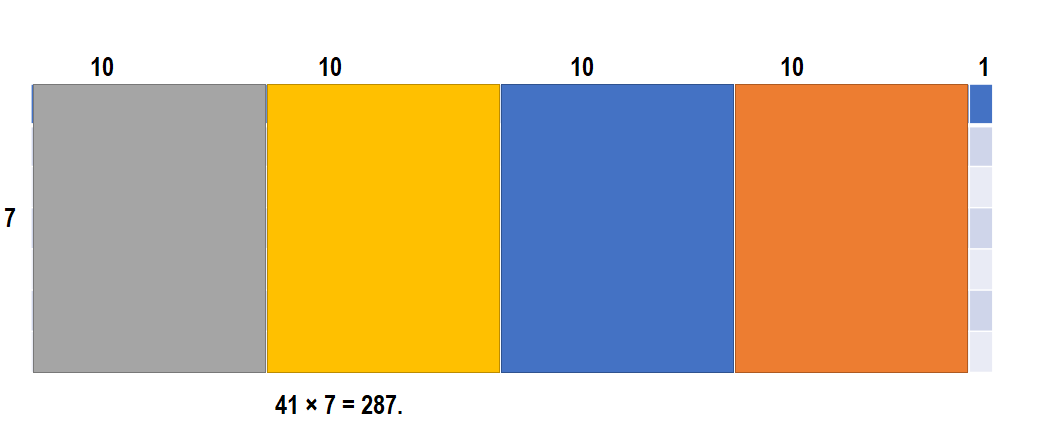Explanation:
Number of rows = 7.
Number of columns = 41.
Product = Number of rows  × Number of columns
= 7 × 41
= (7 × 10) + (7 × 10) + (7 × 10) + (7 × 10) + (7 × 1)
= 70 + 70 + 70 + 70 + 7
= 140 +  70 + 70 + 7
= 210 + 70 + 7
= 280 + 7
= 287.

Problem Solving
Use the table for 8-13.
Question 8.
Representations What is the cost of 3 Purple Lilac trees?Cost of 3 Purple Lilac trees = $87. Explanation: Number of Purple Lilac trees = 3. Cost of each Purple Lilac trees =$29.
Cost of 3 Purple Lilac trees = Number of Purple Lilac trees × Cost of each Purple Lilac trees
= 3 × $29 = (3 ×$10) + (3 × $10) + (3 ×$9)
= $30 +$30 + $27 =$60 + $27 =$87.

Question 9.
Multi-Step What is the cost of 2 Magnolia trees and 1 American Redbud?
Cost of 2 Magnolia trees and 1 American Redbud = $137. Explanation: Cost of each Magnolia trees =$59.
Number of Magnolia trees = 2.
Cost of 2 Magnolia trees = Cost of each Magnolia trees × Number of Magnolia trees
= $59 × 2 = ($10 × 2) + ($10 × 2) + ($10 × 2) + ($10 × 2) + ($10 × 2) + ($9 × 2) =$20 + $20 +$20 + $20 +$20 + $18 =$40 + $20 +$20 + $20 +$18
= $60 +$20 + $20 +$18
= $80 +$20 + $18 =$100 + $18 =$118.
Cost of each American Redbud = $19. Number of American Redbud = 1. Cost of 1 American Redbud = Cost of each American Redbud × Number of American Redbud =$19 × 1
= $19. Cost of 2 Magnolia trees and 1 American Redbud = Cost of 2 Magnolia trees + Cost of 1 American Redbud =$118 + $19 =$137.

Question 10.
H.O.T. Multi-Step What is the difference in cost of 3 Crepe Myrtle trees and 4 Purple Lilacs?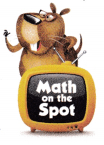$101 is the difference in cost of 3 Crepe Myrtle trees and 4 Purple Lilacs. Explanation: Cost of each Purple Lilac trees =$29.
Actual cost of 4 Purple Lilacs = Cost of each Purple Lilac trees × 4
= $29 × 4 = ($10 × 4) + ($10 × 4) + ($9 × 4)
= $40 +$40 + $36 =$80 + $36 =$116.
Discounted for each Purple Lilacs  = $25. Discounted for each Purple Lilacs =$25 × 4
= ($10 × 4) + ($10 × 4) + ($5 × 4) =$40 + $40 +$20
= $80 +$20
= $100. Cost of 4 Purple Lilacs = Cost of 4 Purple Lilacs – Discounted for each Purple Lilacs =$116 – $100 =$16.
Cost of each Crepe Myrtle trees = $39. Actual cost of 3 Crepe Myrtle trees = Cost of each Crepe Myrtle trees × 3 =$39 × 3
= ($10 × 3) + ($10 × 3) + ($10 × 3) + ($9 × 3)
= $30 +$30 + $30 +$27
= $60 +$30 + $27 =$90 + $27 =$117.
Difference in cost of 3 Crepe Myrtle trees and 4 Purple Lilacs = Actual cost of 3 Crepe Myrtle trees – Cost of 4 Purple Lilacs
= $117 –$16
= $101. Question 11. Pose a Problem Look back at Problem 10. Write a similar problem by changing the number and type of trees. Then solve the problem. Answer: Question: What is the difference in cost of 3 Magnolia trees and 2 American Redbud? Solution:$139 is the difference in cost of 3 Magnolia trees and 2 American Redbud.

Explanation:
Cost of Magnolia trees = $59. Cost of 3 Magnolia trees = 3 ×$59
= (3 × 10) + (3 × 10) + (3 × 10) + (3 × 10) + (3 × 10) + (3 × 9)
= 30 + 30 + 30 + 30 + 30 + 27
= 60 + 30 + 30 + 30 + 27
= 90 + 30 + 30 + 27
= 120 + 30 + 27
= 150 + 27
= $177. Cost of American Redbud =$19.
Cost of  2 American Redbud = 2 × $19 = (2 × 10) + (2 × 9) = 20 + 18 =$38.
Difference:
Cost of 3 Magnolia trees – Cost of  2 American Redbud
= $177 –$38
= $139. Question 12. H.O.T. Multi-Step How much will you save by buying 6 American Redbuds at the discounted price instead of at the regular price? Answer:$24 will be saved by buying 6 American Redbuds at the discounted price instead of at the regular price.

Explanation:
Cost of American Redbuds = $19. Regular cost of 6 American Redbuds = 6 ×$19
= (6 × 10) + (6 × 9)
= 60 + 54
= $114. Cost of discounted price per tree American Redbuds =$15.
Cost of discounted price per tree 6 American Redbuds = 6 × $15 = (6 × 10) + (6 × 5) = 60 + 30 =$90.

Cost of 6 American Redbuds after the discounted price = Regular cost of 6 American Redbuds – Cost of discounted price per tree 6 American Redbuds
= $114 –$90
= $24.Question 13. write Math What’s the Question? Mario multiplied by 4 and used the information in the table. The answer is$204.
No, answer is not correct because Mario multiplied by 4 and used the information in the table, the answer is $84 not$204.

Explanation:Cost of Magnolia Grandiflora = $59. Cost of America Redbud =$19.
Cost of Zuni Crepe Myrtle = $39. Cost of Purple Lilac =$29.
Actual price of all trees = Cost of Magnolia Grandiflora + Cost of America Redbud + Cost of Zuni Crepe Myrtle + Cost of Purple Lilac
= $59 +$19 + $39 +$29
= $78 +$39 + $29 =$117 +$29 =$146.
Discounted price per each Magnolia Grandiflora tree (4 or more) = $51. Discounted price per each America Redbud (4 or more) =$15.
Discounted price per each Zuni Crepe Myrtle (4 or more) = $34. Discounted price per each Purple Lilac (4 or more) =$25.
Total discounted price of all trees (4 or more) = Discounted price per each Magnolia Grandiflora tree (4 or more) + Discounted price per each America Redbud (4 or more) + Discounted price per each Zuni Crepe Myrtle (4 or more) + Discounted price per each Purple Lilac (4 or more)
= $51 +$15 + $34 +$25
= $66 +$34 + $25 =$100 + $25 =$125.
Mario multiplied by 4.
=> Total price of all trees = Actual price of all trees – Total discounted price of all trees (4 or more)
= ($146 –$125) × 4
= $21 × 4 = ($10 × 4) + ($10 × 4) + ($1 × 4)
= $40 +$40 + $4 =$80 + $4 =$84.

Fill in the bubble for the correct answer choice.
Question 14.
There are 26 teams in the spring baseball league. Each team needs 5 parent volunteers. How many volunteers do the teams need?
(A) 31
(B) 30
(C) 100
(D) 130
Number of volunteers do the teams needs = 130.
(D) 130.

Explanation:
Number of teams in the spring baseball league = 26.
Number of parent volunteer each team needs = 5.
Number of volunteers do the teams needs = Number of teams in the spring baseball league × Number of parent volunteer each team needs
= 26 × 5
= (10 × 5) + (10 × 5) + (6 × 5)
= 50 + 50 + 30
= 100 + 30
= 130.

Question 15.
Use Tools Richmond Elementary School has 5 reading classes. Each class sends 19 students to the school spelling bee. How many students are in the school spelling bee?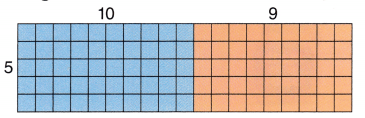(A) 24
(B) 45
(C) 95
(D) 545
Number of students  are in the school spelling bee = 95.
(C) 95.

Explanation:
Number of reading classes Richmond Elementary School has = 5.
Number of students each class sends to the school spelling bee = 19.
Number of students  are in the school spelling bee = Number of reading classes Richmond Elementary School has  × Number of students each class sends to the school spelling bee
= 5 × 19
= (5 × 10) + (5 × 9)
= 50 + 45
= 95.

Question 16.
Multi-Step Kyle lives 17 miles away from Main City Park, He goes to the park and then back home 3 times each week. How many miles does Kyle travel to and from Main City Park each week?(A) 102 miles
(B) 17 miles
(C) 34 miles
(D) 912 miles
Number of miles Kyle travel to and from Main City Park each week = 102.
(A) 102 miles.

Explanation:
Number of miles away from Main City Park Kyle lives = 17.
Number of times he goes to the park and then back home each week = 3.
Number of miles Kyle travel to and from Main City Park each week = (2 × Number of miles away from Main City Park Kyle lives) × Number of times he goes to the park and then back home each week
= (2 × 17) × 3
=[(10 × 2) + (7 × 2)] × 3
= (20 + 14) × 3
= 34 × 3
= (3 × 10) + (3 × 10) + (3 × 10)  + (3 × 4)
= 30 + 30 + 30 + 12
= 60 + 30 + 12
= 90 + 12
= 102.

Texas Test Prep
Question 17.
Mr. Perry bought 6 gallons of juice for a class party. Each gallon has 16 cups. ¡f3 cups of juice were left over, how many cups did students drink?
(A) 48 cups
(B) 9 cups
(C) 93 cups
(D) 13 cups
Number of cups students drink = 93.
(C) 93 cups.

Explanation:
Number of gallons of juice for a class party Mr. Perry bought = 6.
Number of cups each gallon has = 16.
Number of cups of juice were left over = 3.
Number of cups students drink = (Number of gallons of juice for a class party Mr. Perry bought × Number of cups each gallon has) – Number of cups of juice were left over
=(6 × 16) – 3
= [(6 × 10) + (6 × 6)] – 3
= (60 + 36)- 3
= 96 – 3
= 93.

### Texas Go Math Grade 3 Lesson 9.4 Homework and Practice Answer Key

Find the product.
Question 1.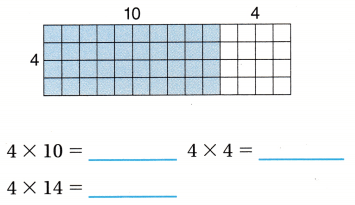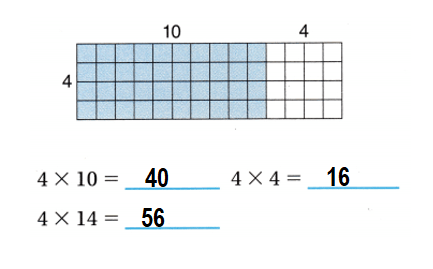Explanation:
Number of rows = 4.
Number of columns = 14.
Product =  Number of columns × Number of rows
= 14 × 4
= (10 × 4) + (4 × 4)
= 40 + 16
= 56.

Question 2.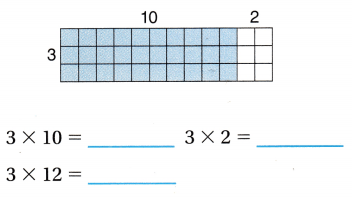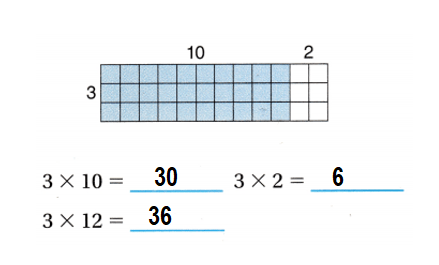Explanation:
Number of columns = 12.
Number of rows = 3.
Product =  Number of columns × Number of rows
= 12 × 3
= (10 × 3) + (2 × 3)
= 30 + 6
= 36.

Find the product. Draw an array to help.

Question 3.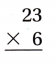Product = 23 × 6 = 138.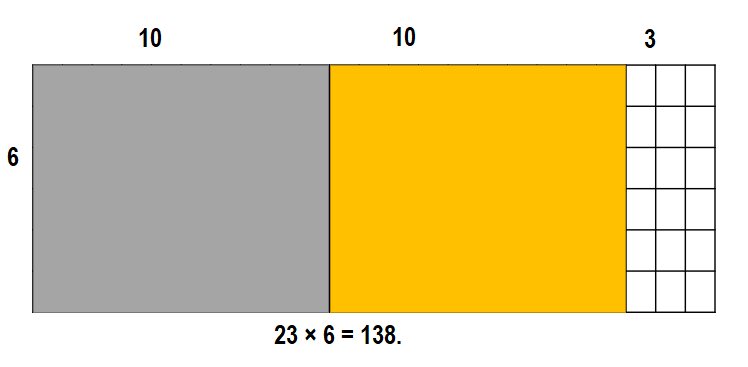Explanation:
Number of columns = 23.
Number of rows = 6.
Product =  Number of columns × Number of rows
= 23 × 6
= (10 × 6) + (10 × 6) + (3 × 6)
= 60 + 60 + 18
= 120 + 18
= 138.

Question 4.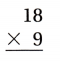Product =  18 × 9 = 162.Explanation:
Number of columns = 18.
Number of rows = 9.
Product =  Number of columns × Number of rows
= 18 × 9
= (10 × 9) + (8 × 9)
= 90 + 72
= 162.

Question 5.Product = 32 × 4 = 128.Explanation:
Number of columns = 32.
Number of rows = 4.
Product =  Number of columns × Number of rows
= 32 × 4
= (10 × 4) + (10 × 4) + (10 × 4) + (2 × 4)
= 40 + 40 + 40 + 8
= 80 + 40 + 8
= 120 + 8
= 128.

Question 6.Product: 75 × 3 = 225.Explanation:
Number of columns = 75.
Number of rows = 3.
Product =  Number of columns × Number of rows
= 75 × 3
= (10 × 3) + (10 × 3) + (10 × 3) + (10 × 3) +(10 × 3) + (10 × 3) +(10 × 3) + (5 × 3)
= 30 + 30 + 30 + 30 + 30 + 30 + 30 + 15
= 60 + 30 + 30 + 30 + 30 + 30 + 15
= 90 + 30 + 30 + 30 + 30 + 15
= 120 + 30 + 30 + 30 + 15
= 150 + 30 + 30 + 15
= 180 + 30 + 15
= 210 + 15
= 225.

Problem Solving
Use the table for 7-9.
Question 7.
If a cow eats 43 pounds of feed in one week, how many pounds do 7 cows eat?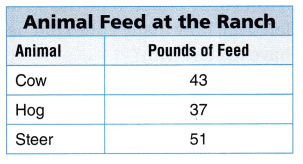Number of pounds of feed in one week 7 cows eats = 301.

Explanation:
Number of pounds of feed in one week a cow eats = 43.
Number of cows = 7.
Number of pounds of feed in one week 7 cows eats = Number of pounds of feed in one week a cow eats × Number of cows
= 43 × 7
= (10 × 7) + (10 × 7) + (10 × 7) + (10 × 7) + (3 × 7)
= 70 + 70 + 70 + 70 + 21
= 140 + 70 + 70  + 21
= 210 + 70 + 21
= 280 +  21
= 301.

Question 8.
There are 3 female hogs and 5 male hogs. How many pounds of feed do they eat altogether?
Number of pounds of feed they eat altogether = 296.

Explanation:
Number of female hogs = 3.
Number of male hogs = 5.
Number of pounds hogs eat = 37.
Number of pounds of feed they eat altogether = (Number of female hogs + Number of male hogs) Number of pounds hogs eat
= (3 + 5) 37
= 8 × 37
= (8 × 10) + (8 × 10) + (8 × 10) + (8 × 7)
= 80 + 80 + 80 + 56
= 160 + 80 + 56
= 240 + 56
= 296.

Question 9.
How many more pounds of feed do 5 steer eat than 5 cows?
40 more pounds of feed 5 steer eat than 5 cows.

Explanation:
Number of steer = 5.
Number of cows = 5.
Number of pounds steer eat = 51.
Number of pounds cows eat = 43.
Total number of pounds 5 steer eat = Number of steer × Number of pounds steer eat
= 5 × 51
= (5 × 10) + (5 × 10) + (5 × 10) + (5 × 10) + (5 × 10) + (5 × 1)
= 50 + 50 + 50 + 50 + 50 + 5
= 100 + 50 + 50 + 50 + 5
= 150 + 50 + 50 + 5
= 200 + 50 + 5
= 250 + 5
= 255.
Total number of pounds 5 cows eat = Number of cows × Number of pounds cows eat
= 5 × 43
= (5 × 10) + (5 × 10) + (5 × 10) + (5 × 10) + (5 × 3)
= 50 + 50 + 50 + 50 + 15
= 100 + 50 + 50 + 15
= 150 + 50 + 15
= 200 + 15
= 215.
Difference:
Total number of pounds 5 steers eat – Total number of pounds 5 cows eat
= 255 – 215
= 40.

Texas test Prep
Lesson Check
Question 10.
The soccer league has 28 teams. Each team has 9 players. How many players are there in all?
(A) 72
(B) 152
(C) 289
(D) 252
Number of players are there in all = 252.
(D) 252.

Explanation:
Number of teams soccer league has = 28.
Number of players each team has = 9.
Number of players are there in all = Number of teams soccer league has × Number of players each team has
= 28 × 9
= (10 × 9) + (10 × 9) + (8 × 9)
= 90 + 90 + 72
= 180 + 72
= 252.

Question 11.
An apple orchard has 8 rows of trees. Each row has 34 trees. How many trees are in the orchard?
(A) 272
(B) 332
(C) 172
(D) 348
Number of trees are in the orchard = 272.
(A) 272.

Explanation:
Number of rows of trees an apple orchard has = 8.
Number of trees each row has = 34.
Number of trees are in the orchard = Number of rows of trees an apple orchard has × Number of trees each row has
= 8 × 34
= (8 × 10) + (8 × 10) + (8 × 10) + (8 × 4)
= 80 + 80 + 80 + 32
= 160 + 80 + 32
= 240 + 32
= 272.

Question 12.
On a school trip, there are 4 buses of students. Each bus holds 58 students. How many students go on the school trip?
(A) 458
(B) 132
(C) 532
(D) 232
Number of students go on the school trip = 232.
(D) 232.

Explanation:
Number of buses of students on a school trip = 4.
Number of students each bus holds = 58.
Number of students go on the school trip = Number of buses of students on a school trip × Number of students each bus holds
= 4 × 58
= (4 × 10) + (4 × 10) + (4 × 10) + (4 × 10) + (4 × 10) + (4 × 8)
= 40 + 40 + 40 + 40 + 40 + 32
= 80 + 40 + 40 + 40 + 32
= 120 + 40 + 40 + 32
= 160 + 40 + 32
= 200 + 32
= 232.

Question 13.
A train travels 47 miles between two cities. If the train makes 4 trips in one day, how many miles does it travel?
(A) 167 miles
(B) 128 miles
(C) 188 miles
(D) 168 miles
Number of miles it travel = 188.
(C) 188 miles.

Explanation:
Number of miles between two cities a train travels = 47.
Number of trips in one day a train makes = 4.
Number of miles it travel = Number of miles between two cities a train travels × Number of trips in one day a train makes
= 47 × 4
= 188.

Question 14.
Multi-Step Luz makes an array of 6 rows of 27 cubes. If he has 9 cubes left over, how many cubes did he have to start with?
(A) 171
(B) 243
(C) 162
(D) 153
(A) 171.

Explanation:
Number of rows Luz makes an array = 6.
Number of cubes = 27.
Number of cubes left over = 9.
Number of cubes he have to start with = (Number of rows Luz makes an array × Number of cubes ) + Number of cubes left over
= (6 × 27) + 9
= 162 + 9
= 171.

Question 15.
Multi-Step There are 8 trays of sandwiches at a picnic. Each tray holds 22 sandwiches. If 12 sandwiches are left over, how many sandwiches were eaten?
(A) 176
(B) 164
(C) 188
(D) 174